Mathematics, Grade 2, Term 3, Test 1
This test is CAPS aligned and could be used for revision purposes in September. This is a self-marking test and the results will be available after submission. The setting of this paper by volunteering teachers is appreciated.
What is your name?
Optional
What the name of your school?
What is the name of your province?
Write the number name for 74
1 point
Decompose the following number: 83
1 point
Calculate the following:
6 + 4 = _____
1 point
7 + _____ = 12
1 point
16 - 8 = _____
1 point
8 x 3 = ______
1 point
15 - _____ = 10
1 point
5 x 10 = ______
1 point
Arrange these numbers from smallest to biggest.
1 point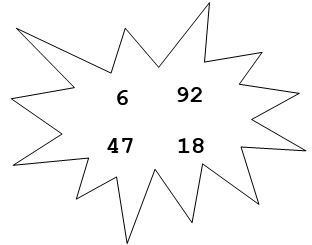Calculate: 24 + 35 = ______
2 points
Half of 46 is ______
2 points
There are 15 apples on the tree. Share them out into 3 baskets. How many apples will be in each basket?
2 points
My mom gave me R20. I bought a hotdog for R10 and a cool drink for R6. How much change will I get?
2 points
The farmer uses a jug to fill up this bucket. Each jug fills the bucket up one line. How many jugs of water will the farmer need to get?
1 point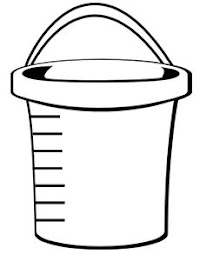How many quarters of this shape are coloured in?
2 points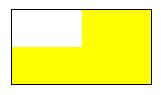Which clock below shows that the time is half past 7?
2 points
Write the name of the given shape?
1 point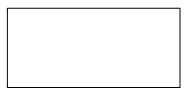Draw a line to match the 3D object to its name:
Choose the correct one from the pictures below.
3 points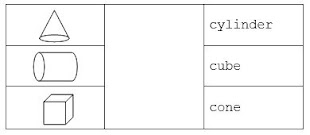Complete the number pattern: 36, 39, 42, _____, _____, _____
2 points
Complete the number pattern: 95, 90, 85, _____, _____, _____
2 points
Complete the pattern:
Choose the correct one from the pictures below.
2 points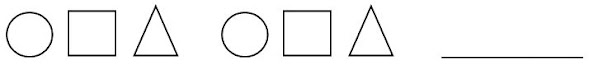This is how the children in the class get to school every day. Study the graph below and answer the questions that follow.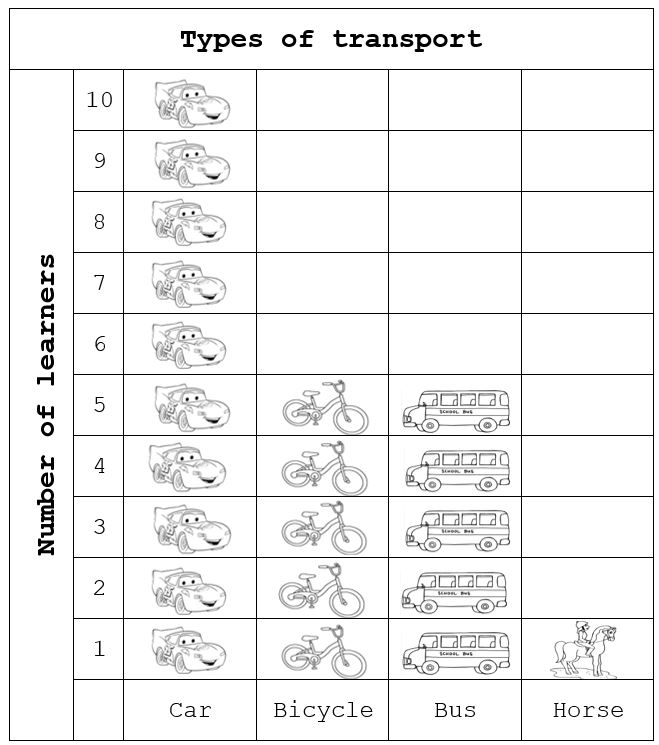Which type of transport do people use the most?
Choose from the list below.
1 point
How many children use a car and bicycle to get to school?
2 points
Never submit passwords through Google Forms.
This content is neither created nor endorsed by Google. Report Abuse - Terms of Service - Privacy Policy